# High School Math : Graphing Absolute Value

## Example Questions

### Example Question #1 : Absolute Value

Find the-intercepts for the graph given by the equation: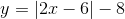Possible Answers: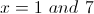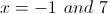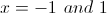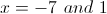Correct answer:Explanation:

To find the-intercepts, we must set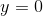.

To solve absolute value equations, we must understand that the absoute value function makes a value positive. So when we are solving these problems, we must consider two scenarios, one where the value is positive and one where the value is negative.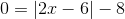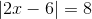Now we must set up our two scenarios: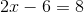and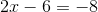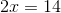and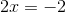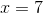and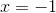### All High School Math Resources